# Basic concepts of functions

A collection of program instructions (statements) for accomplishing a certain function is called a function.
In Go, functions are divided into: custom functions and system functions.

# Basic syntax of functions

``````func 函数名（形参列表）（返回值列表）{

执行语句...
return 返回值列表
}
``````
• Formal parameter list: represents the input of the function
• Sentences in the function: It means to realize a certain function code block.
• A function can have a return value or no return value.

## Case presentation

``````package main
import "fmt"

func getCal(n1 int , n2 int)(int, int){

sum := n1 + n2
sub := n1 - n2

return sum, sub
}

func main(){

var n1 int  = 10
var n2 int  = 20

//调用函数getCal计算两个数的和和差
sum, sub := getCal(n1,n2)
fmt.Println("sum =",sum,"sub =",sub)

}

``````

operation result: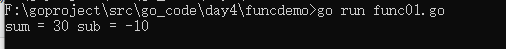# Function call mechanism

Introduction: In order to better understand the calling process of the function, look at two cases and draw a schematic diagram.

## Case demonstration:

Look at the following code, what is the output?

``````package main
import "fmt"

func test(n1 int){

n1 = n1 + 1
fmt.Println("test() n1 =",n1)//输出结果=？
}
func main(){

n1 := 10
//调用函数test
test(n1)
fmt.Println("main() n1 =",n1)//输出结果=？

}

``````

Operation result: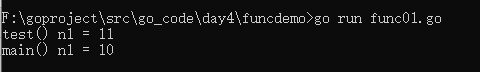Schematic diagram of the bottom layer: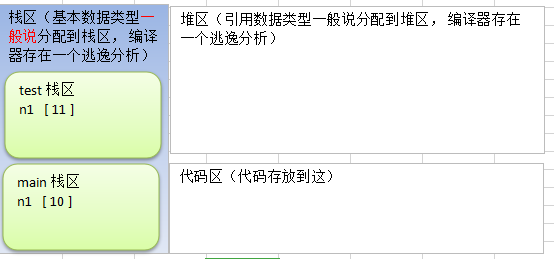Description:

• When a function is called, a new space will be allocated to the function, and the compiler will distinguish this new space from other stack spaces through its own processing.
• In the stack corresponding to each function, the data space is independent and will not be confused
• When a function is called, the program will destroy the stack space corresponding to this function.

# return statement

## Basic grammar and instructions

Go supports returning multiple values, which is not available in other programming languages.
Basic syntax:

``````func 函数名（形参列表）（返回值列表）{

执行语句...
return 返回值列表
}
``````

Description:

• If multiple values ​​are returned, if you want to ignore a certain return value when receiving, you can use the _ symbol to indicate that the placeholder is ignored.
``````package main
import "fmt"

func getCal(n1 int , n2 int)(int, int){

sum := n1 + n2
sub := n1 - n2
return sum, sub
}

func main(){

var n1 int  = 10
var n2 int  = 20

//调用函数getCal计算两个数的和和差
sum, sub := getCal(n1,n2)
//接收两个数的和,
sum1, _ := getCal(n1,n2)
fmt.Println("sum =",sum,"sub =",sub)
fmt.Println("sum1 =",sum1)
}
``````
• If there is only one return value, (return value list) can not write ()
``````package main
import "fmt"

func test(n1 int) int{

n1 = n1 + 1
return  n1
}
func main(){

n1 := 10

res := test(n1)
fmt.Println("res =",res)

}

``````

# Recursive function call

## basic introduction

A function calls itself in the function body, we call it recursive call.

## Quick start

### Case

``````package main
import "fmt"

func test(n int){

if n > 2{

n--
test(n)
}
fmt.Println("n =",n)
}

func main(){

test(4)//通过分析来看函数递归调用的特点

}
``````

### Analysis chart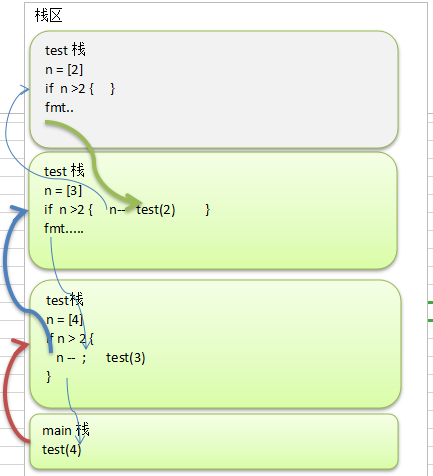Output result: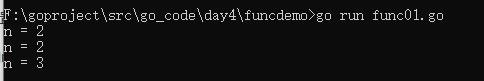### Description

The stack is a first-in-last-out data structure. When the value of n is 2, the condition is not met, the output statement is executed, 2 is output, and then it returns (pop). At this time, n=3, and the condition is met, n–after , Is 2, output 2, in the next pop, the value after n– is 3, then output 3.

## Summary of recursive calls

1. When a function is executed, a new protected independent space is created.
2. The local variables of the function are independent and will not affect each other.
3. Recursion must approach the exit recursion condition, otherwise it will be infinite recursion.
4. When a function is executed, or when it encounters a return, it means return. Whoever calls it will return the result to whoever is called. At the same time, when the function is executed or returns, the function itself will also be destroyed by the system.

# Notes on the use of functions

1. There can be multiple function parameter lists and multiple return value lists.
2. The data types of the formal parameter list and the return value list can be value types and reference types.
3. The naming of the function follows the identifier naming convention. The first letter cannot be a number, and the first letter is capitalized. This function can be used by this package file and other package files, similar to public, the first letter is lowercase, and can only be used by this package file, but other package files cannot Use, similar to private.
4. The variables in the function are local and do not take effect outside the function.
5. Basic data types and arrays are passed by value by default, that is, value copy is performed. Modifications within the function will not affect.
``````package main
import "fmt"

func test(n string){

n = n + "321"
fmt.Println("n =",n)
}

func main(){

var name string = "casey"
test(name)
fmt.Println("name =",name)
}
``````

operation result: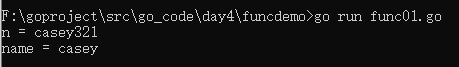1. If you want the variables in the function to modify the variables outside the function (referring to the data type that is passed by value by default), you can pass in the address of the variable &, and manipulate the variable in the function as a pointer.
``````package main
import "fmt"

func test(n *string){

*n = *n + "321"
fmt.Println("*n =",*n)
}

func main(){

var name string = "casey"
test(&name)
fmt.Println("name =",name)
}
``````

Operation result: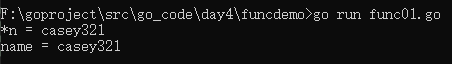schematic diagram: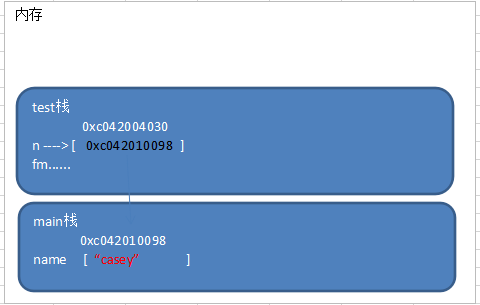1. Go functions do not support function overloading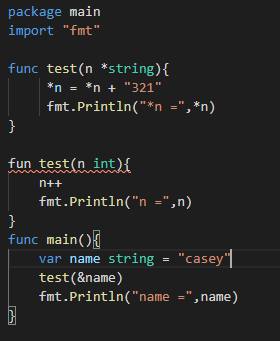2. In Go, a function is also a data type , which can be assigned to a variable, then the variable is a variable of the function type. The function can be called through this variable.
``````package main
import "fmt"

func test(n1 int,n2 int) int {

return n1 + n2
}

func main(){

a := test
fmt.Printf("a的类型为%T,test的类型为%T\n",a ,test)

res := a(10,40)//等价 res := test(10, 40)
fmt.Println("res =",res)
}
``````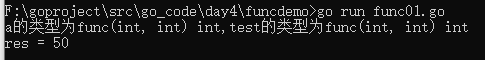1. Function is a data type. In Go, functions can be used as formal parameters and called.
``````package main
import "fmt"

func test(n1 int,n2 int) int {

return n1 + n2
}

func myFunc(funcvar func(int,int) int,num1 int ,num2 int) int{

return funcvar(num1, num2)
}

func main(){

a := test
fmt.Printf("a的类型为%T,test的类型为%T\n",a ,test)

res := myFunc(a,100,20)
fmt.Println("res =",res)
}
``````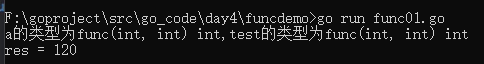1. In order to simplify the definition of data types, Go supports custom data types.
``````基本语法：
type 自定义数据类型名 数据类型//相当于一个别名

type myFunc func(int,int) int
``````
``````package main
import "fmt"

func main(){

type myInt int
var num1 myInt
var num2 int
num1 = 20
//注意：num2 = num1 会报错，go认为myInt和int是两个不同类型
num2 = int(num1)
fmt.Println("num1 =",num1,"num2 =",num2)
}
``````

operation result: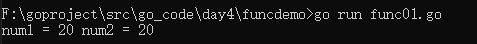1. Support naming function return values
``````package main
import "fmt"

func getSumAndSub(n1 int,n2 int)(sum int, sub int) {

sum = n1 + n2
sub = n1 - n2
return
}

func main(){

a1, b1 := getSumAndSub(15,30)
fmt.Printf("sum = %v,sub = %v\n",a1,b1)
}
``````

operation result: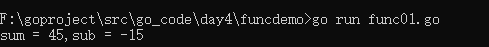1. Use _ identifier, ignore return value
``````func main(){

a1, _ := getSumAndSub(15,30)
fmt.Printf("sum = %v\n",a1)
}
``````
1. Go supports variable parameters
``````package main
import "fmt"

func getSum(n1 int,args...int) int {

sum := n1
for i := 0; i < len(args); i++{

sum += args[i]
}
return sum
}

func main(){

sum := getSum(10,0,5,20,-5)
fmt.Printf("sum = %v\n",sum)
}
``````

Operation result: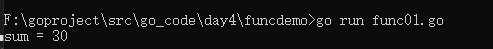Description:

• args is a slice slice, and each value can be accessed through args[index].
• If there are variable parameters in the formal parameter list of a function, the variable parameters need to be placed at the end of the formal parameter list.

# Function defer

## Why do you need defer

In functions, programmers often need to create resources (such as database connections, file handles, etc.). In order to release resources in time after the function is executed, the designer of Go provides defer ( delay mechanism )

## Case

``````package main
import "fmt"

func getSum(n1 int,n2 int) int {

//当执行到defer是，暂不执行，会将defer后面的语句压入到独立的栈中，
//当函数执行完毕后，再从defer栈，按照先进后出的方式出栈
defer fmt.Println("ok1 n1 =",n1)
defer fmt.Println("ok2 n2 =",n2)
sum := n1 + n2
fmt.Println("ok3 sum =",sum)
return sum
}

func main(){

sum := getSum(10,-5)
fmt.Printf("sum = %v\n",sum)
}
``````

operation result: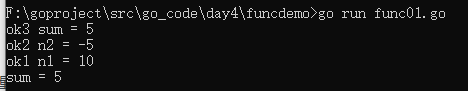## Note for defer

• When go executes to a defer, the statement after defer will not be executed immediately, but the statement after defer is pushed onto the stack (for ease of understanding, temporarily call the stack as the defer stack), and then continue to execute the next function of the function Statement.
• After the function is executed, in the defer stack, the statements are taken out from the top of the stack and executed in a first-in-last-out manner.

## Defer application scenarios

The main value of defer is that when the function is executed, it can release the resources created by the function in time. Look at the following example simulation code: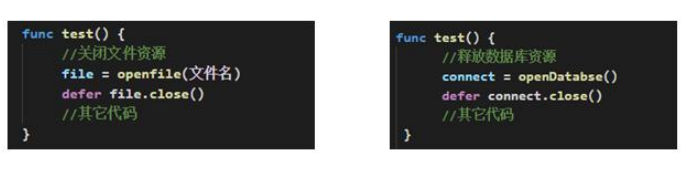This mechanism is very concise, and programmers don't need to worry about closing resources at the time.

# Function parameter passing method

## Two delivery methods

The two methods of function parameter transfer are: value transfer and reference transfer. Whether it is value transfer or reference transfer, the copy of the variable is passed to the function. The difference is that the value transfer is the copy of the value, and the reference transfer is the address. Generally speaking, the address copy efficiency is high, because the amount of data is small, and the value copy determines the size of the copied data. The larger the data, the lower the efficiency.

## Value type and reference type

Please refer to this blog
1. The default value type is value transfer: variables store values ​​directly, and memory is usually allocated on the stack.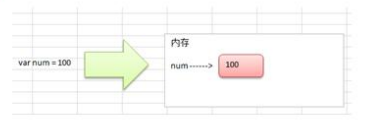2. The default of the reference type is to pass by reference: the variable stores an address, and the space corresponding to this address actually stores the data (value). The memory is usually allocated on the heap. When there is no variable referencing this address, the data corresponding to the address The space becomes a garbage, which is recycled by Gc.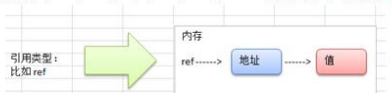3. If you want the variables in the function to modify the variables outside the function, you can pass in the address of the variable &, and manipulate the variables in the function as a pointer. Seen from the effect, it is similar to the reference.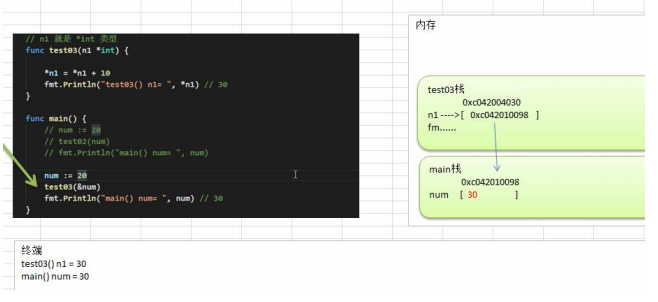### Guess you like

Origin blog.csdn.net/weixin_44736475/article/details/113936224
Recommended
Ranking
Daily